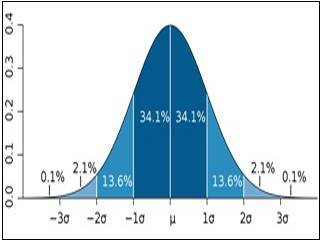15.075J | Fall 2011 | Undergraduate
Statistical Thinking and Data Analysis
Course Description
This course is an introduction to statistical data analysis. Topics are chosen from applied probability, sampling, estimation, hypothesis testing, linear regression, analysis of variance, categorical data analysis, and nonparametric statistics.
Course Info
Instructors
Departments
Topics
Learning Resource Types
assignment_turned_in Problem Sets with Solutions